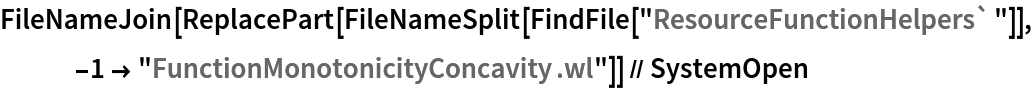Function Repository Resource:

# FunctionMonotonicity

Compute the regions on which an expression is monotonic

Contributed by: Wolfram|Alpha Math Team
 ResourceFunction["FunctionMonotonicity"][f,x] returns an association of information about where f is increasing, decreasing or constant with respect to x. ResourceFunction["FunctionMonotonicity"][f,x,property] returns a specific property related to whether f is increasing, decreasing or constant with respect to x.

## Details

ResourceFunction["FunctionMonotonicity"] expects f to be a univariate expression in terms of x, similar to what might be entered into Plot.
ResourceFunction["FunctionMonotonicity"] returns regions on which f is strictly monotonic, that is, f is strictly increasing on an interval A if for all {x,y}A such that x<y, it is the case that f[x]<f[y].
The input property can be any of All, "Increasing", "Decreasing", "Constant", "Regions", "Plot" or "NumberLine", and defaults to "Regions".

## Examples

### Basic Examples (2)

Compute the regions on which a curve is increasing, decreasing and constant:

 In:=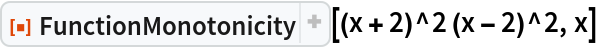Out=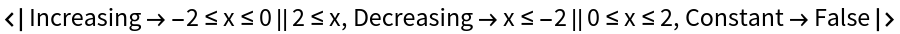Show plots as well as the regions:

 In:=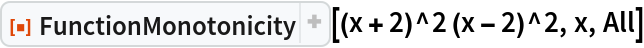Out=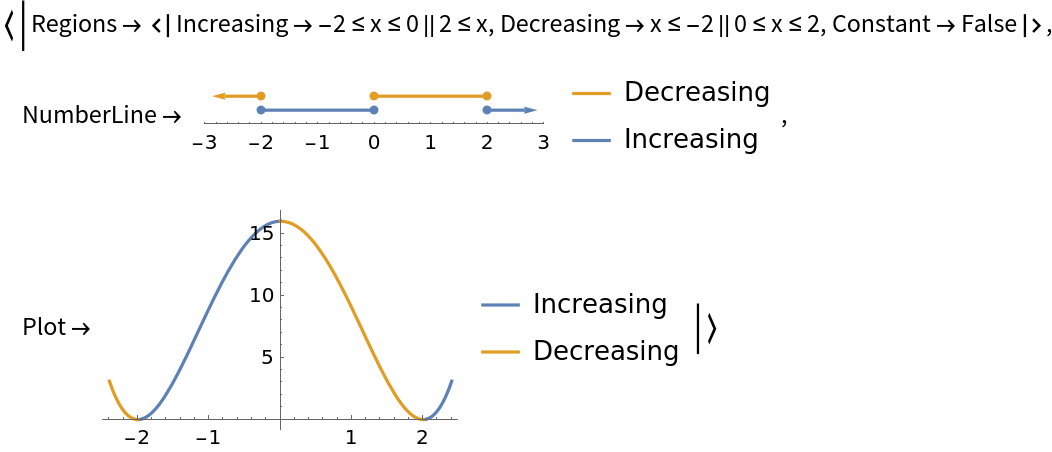### Scope (3)

FunctionMonotonicity returns strict regions of increasing and decreasing, as can be seen in this piecewise expression:

 In:=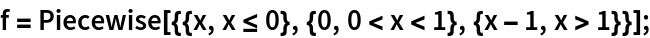In:=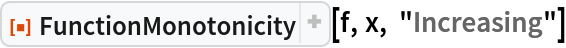Out=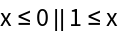In:=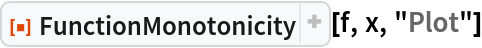Out=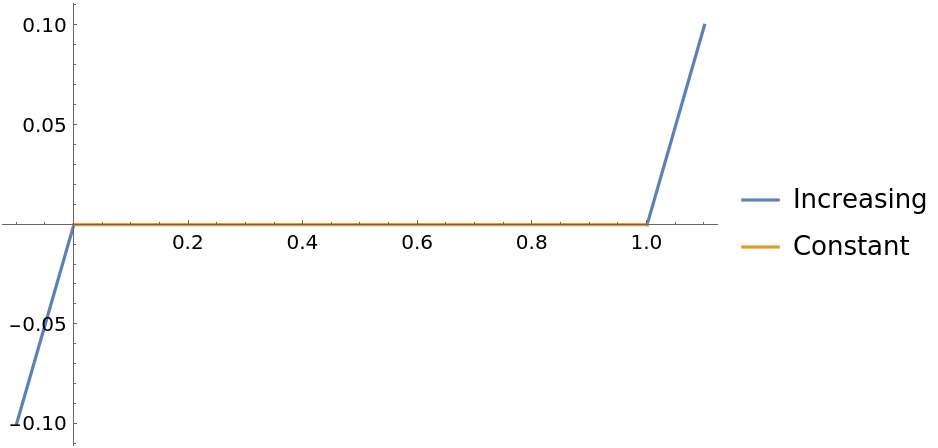Return the "NumberLine" property to visualize the regions directly:

 In:=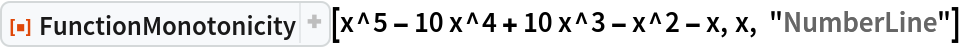Out=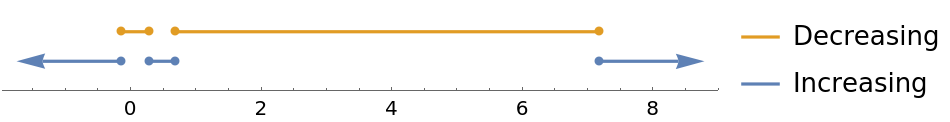Or return the "Plot" property to visualize the regions on the plot of the curve:

 In:=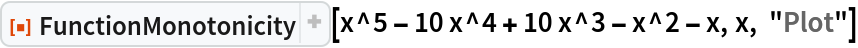Out=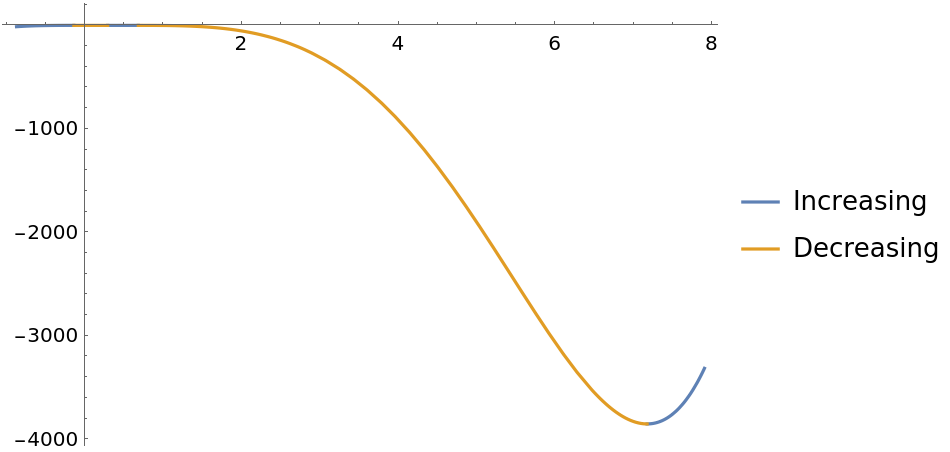Note that a curve can be both increasing and decreasing at a given point:

 In:=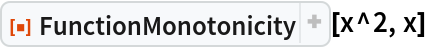Out=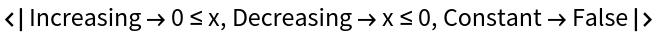In this case, 0 is a member of both the increasing and decreasing regions. To test that this is true against the definition of a curve being strictly increasing for x0, use Resolve:

 In:=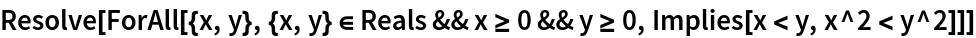Out=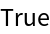Likewise to test if the curve is decreasing for x0:

 In:=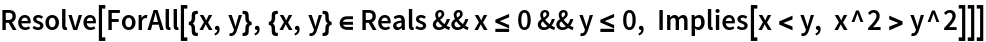Out=### Possible Issues (3)

Working with the absolute value function Abs may return unexpected results:

 In:=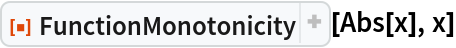Out=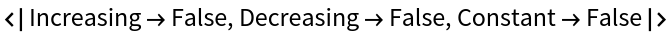This is due to the assumption of Complex inputs to Abs in comparison to RealAbs:

 In:=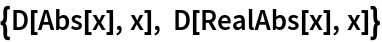Out=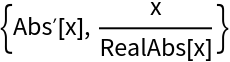Using RealAbs returns the expected results:

 In:=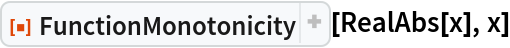Out=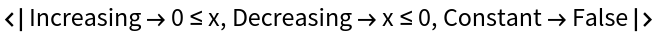## Publisher

Wolfram|Alpha Math Team

## Version History

• 3.0.1 – 27 March 2023
• 3.0.0 – 23 March 2023
• 2.0.0 – 23 March 2023
• 1.0.0 – 29 September 2020

## Author Notes

To view the full source code for FunctionMontonicity, run the following code:

 In:=